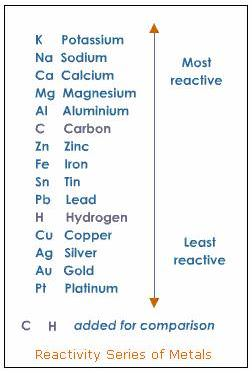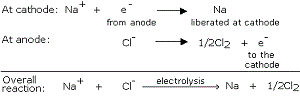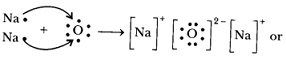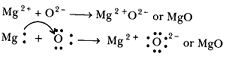### Metals and Non-metals - Test Papers

CBSE Test Paper-01

Chapter 03 Metals and Non Metals

1. Metal always found in free state is? (1)

1. Sodium
2. Lithium
3. Copper
4. Gold
2. Roasting is a method of heating ore: (1)

1. In the absence of water.
2. In the presence of water.
3. In the absence of air.
4. In the presence of air.
3. Ayush is putting ${\mathrm{H}}_{2}\mathrm{S}{\mathrm{O}}_{4}$ in the test tube containing water whereas Piyush is putting water in the lest tube containing ${\mathrm{H}}_{2}\mathrm{S}{\mathrm{O}}_{4}$. Which of the two is likely to face danger? (1)

1. Piyush
2. Ayush
3. None of the two
4. Both Ayush and Piyush
4. The group of metals which do not react with oxygen: (1)

1. All of these
2. Na, Cs
3. Au, Ag
4. Pt, Cu
5. Magnesium imparts: (1)

1. Dazzling white colour flame
2. Yellowish orange colour flame
3. None of these
4. Brick red colour flame
6. A non-metal X exists in two different forms Y and Z. Y is hardest natural substance, whereas Z is a good conductor of electricity. Identify X, Y arid Z. (1)

7. Why are metals reducing agents where as non metals are oxidizing agents? (1)

8. Name two metals which will displace hydrogen from dilute acids, and two metals which will not? (1)

9. Name two metals that do not react with water at all. (1)

10. State the property utilised in the following: (3)

1. Graphite in making electrodes.
2. Electrical wires are coated with Polyvinyl Chloride (PVC) or a rubber-like material.
3. Metal alloys are used for making bells and strings of musical instruments.
11. Choose from the following metals to answer the questions below: (3)
Aluminium, calcium, copper, iron, magnesium, potassium, nickel and zinc. Name a metal which

1. is manufactured by the electrolysis of its molten oxide.
2. is used to galvanise iron
3. is alloyed with zinc to make brass.
4. reacts with aqueous copper (II) sulphate to give a pink solid.
5. does not react with cold water.
12. How will you show that silver is less reactive than copper? (3)

13. Why metals replace hydrogen from dilute acids, whereas non-metals do not? (3)

1. Distinguish between ionic and covalent compounds under the following properties: (5)
1. Strength of forces between constituent elements
2. Solubility of compounds in water
3. Electrical conduction in substances
2. Explain how the following metals are obtained from their compounds by the reduction process:
1. Metal M which is in the middle of the reactivity series.
2. Metal N which is high up in the reactivity series. Give one example of each type.

1. Write the electron-dot structures for sodium, oxygen and magnesium.
2. Show the formation of $N{a}_{2}O$and $MgO$by the transfer of electrons.
3. What are the ions present in these compounds? (5)

CBSE Test Paper-01
Chapter 03 Metals and Non Metals

1. Gold
Explanation: Gold, platinum, copper, silver are few metals which can be found in free state, because they are unreactive in normal conditions with air, water and other chemicals.
1. In the presence of air.
Explanation: Roasting is heating of an ore in a regular supply of air in a furnace.
1. Piyush
Explanation: Piyush is likely to face danger. Addition of water to concentrated sulphuric acid is an exothermic reaction and can lead to explosions. Water should not be poured into a container containing an acid.
1. Au, Ag
Explanation: Gold and Silver do not react with oxygen. They are less reactive metals and lie at the bottom of the reactivity series.
1. Dazzling white colour flame
Explanation: Magnesium burns in air with a dazzling white flame.
1. The non-metal X is carbon (C). Y and Z are the allotropes of carbon (different physical forms of carbon). Y is diamond because diamond is the hardest natural substance known and Z is graphite which is a good conductor of electricity.

2. Metals have a tendency to donate electrons and get oxidized. Thus, they are reducing agents.
Non- metals on the other hand have a tendency to gain electrons and get reduced. Therefore, they are oxidizing agents.

3. Sodium and calcium, being more reactive than hydrogen can displace hydrogen from dilute acids.
Copper and silver, being less reactive than hydrogen cannot displace hydrogen from dilute acids.

1. Graphite in an allotrope of carbon which is a good conductor of electricity because of presence of free electron and it is cheap, insoluble in water, do not react with acids and bases (non-corrosive). Due to these properties, it is used in making electrodes.
2. Polyvinyl Chloride (PVC) or a rubber-like material are insulators means they are bad conductors of electricity and hence do not allow electrons to flow. Hence, these are used in coating the electrical wires.
3. Metal alloys are used for making bells and strings of musical instruments because they are sonorous.
1. The metal which is manufactured by the electrolysis of its molten oxide is Aluminium.
2. Zinc is used to galvanise iron, because it is more reactive.
3. Copper is alloyed with zinc to make brass.
4. Iron reacts with aqueous copper (II) sulphate to give a pink solid.
5. Iron has no reaction with cold water but it reacts with steam.
5. In activity series silver is placed below the copper. hence copper is more reactive than silver.The more reactive element can displace the less reactive element from its solution.
take two test tubes in test tube -1 take silver Sulphate solution(white in color) & in test tube-2 take copper Sulphate solution (blue in color). Add copper turnings to test tube -1 and add silver piece to test tube -2 keep the test tubes undisturbed for 15 minutes. After sometime observe the color changes in both test tubes. The color in test tube – 1 changed from white to blue and there is no color change in test tube-2.this indicates in test tube-1 copper displaces silver from its silver Sulphate solution forms copper Sulphate. And in test tube- 2 no displacement reaction takes place. Hence from this experiment we are proving that copper is more reactive than silver.

6. The reason why non-metals do not displace hydrogen from dilute acids is because unlike metals, non-metals do not have a tendency to lose electrons but to gain electrons. Metals have a tendency to lose electrons. These electrons, which are readily lost by reactive metals like sodium, potassium etc are accepted by hydrogen ions of the acids, reducing them to hydrogen gas (H2)
2H+ + 2e→ H2
However, non-metals do not lose electrons readily, because of which they do not displace hydrogen from acids. Another important point to note is that not all metals will displace hydrogen from acids. Only those metals which are reactive than hydrogen will displace H2 from acid.

1. Ionic compounds have strong force of attraction between the oppositely charged ions (e.g., $N{a}^{+}$ and $C{l}^{-}$), so they are solids. Covalent compounds have weak force of attraction between their molecules, so they are usually liquids or gases.
2. Ionic compounds are soluble in water but covalent compounds are insoluble in water.
3. Ionic compounds conduct electricity when dissolved in water or when melted because they contain ions (charged particles). But, covalent compounds like glucose do not conduct electricity because they do not contain ions.
1. The metal M which is in the middle of the reactivity series (such as iron, zinc, lead, copper, etc.) is moderately reactive. So, for obtaining such metals from their compounds, their sulphides and carbonates (in which they are present in nature) are first converted into their oxides by the process of roasting and calcination respectively. For example,
$\underset{\underset{\left(Sulphideore\right)}{Zinc\phantom{\rule{thinmathspace}{0ex}}Sulphide}}{2ZnS\left(s\right)}+3{O}_{2}\left(g\right)\stackrel{Heat}{⟶}2ZnO\left(s\right)+2S{O}_{2}\left(g\right)$
$\underset{\underset{\left(Carbonate\phantom{\rule{thinmathspace}{0ex}}are\right)}{Zinc\phantom{\rule{thinmathspace}{0ex}}\phantom{\rule{thinmathspace}{0ex}}Carbonate}}{ZnC{O}_{3}\left(s\right)}\stackrel{Heat}{⟶}ZnO\left(s\right)+C{O}_{2}\left(g\right)$
The metal oxide (MO) are then reduced to the corresponding metals by using suitable reducing agents such as carbon. For example, zinc metal from its oxide is obtained as follow:
$\underset{Zinc\phantom{\rule{thinmathspace}{0ex}}\phantom{\rule{thinmathspace}{0ex}}oxide}{ZnO\left(s\right)}\phantom{\rule{thinmathspace}{0ex}}+\phantom{\rule{thinmathspace}{0ex}}C\left(s\right)\phantom{\rule{thinmathspace}{0ex}}\to \underset{Zinc}{Zn\left(s\right)}\phantom{\rule{thinmathspace}{0ex}}+\phantom{\rule{thinmathspace}{0ex}}CO\left(g\right)$
2. The metal N which is high up in the reactivity series (such as sodium, magnesium, calcium, aluminium, etc.), is very reactive and cannot be obtained from its compound by heating with carbon.
Therefore, such metals are obtained by electrolytic reduction of their molten salt. For example, sodium is obtained by the electrolysis of molten sodium chloride ($NaCl$).7. MetalSymbolAtomic numberElectronic configuration K, L, M, NNo. of outermost electronsElectron dot structures
SodiumNa112,8, 11Na.
OxygenO82,66:O::
MagnesiumMg122,8, 22Mg:
1. Formation of $N{a}_{2}O$:
The atomic number of sodium is 11 and it has only one valence electron.
Hence, electronic configuration of ${}_{11}Na$is 2, 8, 1.
The atomic number of oxygen is 8 and it has 6 electrons in its valence shell.
Hence, electronic configuration of ${}_{8}O$is 2, 6.
Sodium has a tendency to lose the valence electron and oxygen has a tendency to gain the electron lost by sodium. Since, sodium can lose only one electron of the valence shell, and oxygen atom needs two electrons to complete its octet in the valence electron, two atoms of sodium combine with one atom of oxygen. By losing valence electron, sodium is changed into $N{a}^{+}$ and by gaining two electrons lost by two sodium atoms, oxygen atom is changed into an oxide anion, ${O}_{2}$ .In this process , both the atoms, sodium and oxygen, obtain the stable electronic configuration of the noble gas neon.
$\underset{2,8,1}{Na}\phantom{\rule{thinmathspace}{0ex}}\to \phantom{\rule{thinmathspace}{0ex}}\underset{2,8}{N{a}^{+}}\phantom{\rule{thinmathspace}{0ex}}+\phantom{\rule{thinmathspace}{0ex}}{e}^{-}$${O}^{2-}$
$\underset{2,6}{O\phantom{\rule{thinmathspace}{0ex}}+\phantom{\rule{thinmathspace}{0ex}}}\phantom{\rule{thinmathspace}{0ex}}2{e}^{-}\phantom{\rule{thinmathspace}{0ex}}\phantom{\rule{thinmathspace}{0ex}}\to \phantom{\rule{thinmathspace}{0ex}}\underset{2,8}{{O}^{2-}}$
$2N{a}^{+}\phantom{\rule{thinmathspace}{0ex}}\phantom{\rule{thinmathspace}{0ex}}+\phantom{\rule{thinmathspace}{0ex}}\phantom{\rule{thinmathspace}{0ex}}{O}^{2}\phantom{\rule{thinmathspace}{0ex}}\phantom{\rule{thinmathspace}{0ex}}\to \phantom{\rule{thinmathspace}{0ex}}\phantom{\rule{thinmathspace}{0ex}}2N{a}^{+}{O}^{2-}\phantom{\rule{thinmathspace}{0ex}}or\phantom{\rule{thinmathspace}{0ex}}N{a}_{2}O$
The oppositely charged sodium ion, $N{a}^{+}{O}^{2-}$ and oxide ion, ${O}^{2-}$ are now held together by electrostatic force of attraction or by ionic or electrovalent bond. $N{a}_{2}O$ is, therefore, an ionic or electrovalent compound.Formation of $MgO$:
The atomic number of magnesium = 12
Its electronic configuration is $\underset{2,}{K,}\phantom{\rule{thinmathspace}{0ex}}\underset{8,}{L,}\underset{2}{M}$
It has two electronic in its outermost shell. So, the magnesium atom donates its 2 valence electrons and forms a stable magnesium ion, ${Mg}^{2+}$ to attain the electronic arrangement of neon atom.
$\underset{2,8,2}{Mg}\phantom{\rule{thinmathspace}{0ex}}\phantom{\rule{thinmathspace}{0ex}}\to \phantom{\rule{thinmathspace}{0ex}}\phantom{\rule{thinmathspace}{0ex}}\underset{2,8}{\overset{2+}{Mg}}\phantom{\rule{thinmathspace}{0ex}}\phantom{\rule{thinmathspace}{0ex}}+\phantom{\rule{thinmathspace}{0ex}}\phantom{\rule{thinmathspace}{0ex}}{2}^{e-}$
The atomic number of oxygen = 8
Electronic configuration = $\underset{2}{K,}\phantom{\rule{thinmathspace}{0ex}}\phantom{\rule{thinmathspace}{0ex}}\underset{6}{L}$
It has 6 electrons in its valence shell. Therefore, it requires 2 more electrons to attain the stable electronic arrangement of neon gas. Thus, oxygen accepts 2 electrons donated by magnesium atom and forms a stable oxide ion, O2-
$\underset{2,6}{O}\phantom{\rule{thinmathspace}{0ex}}\phantom{\rule{thinmathspace}{0ex}}+\phantom{\rule{thinmathspace}{0ex}}\phantom{\rule{thinmathspace}{0ex}}2{e}^{-}\phantom{\rule{thinmathspace}{0ex}}\phantom{\rule{thinmathspace}{0ex}}\to \underset{2,8}{\overset{2-}{O}}$
The oppositely charged magnesium ions, ${Mg}^{2+}$, and oxide ions, are held together by a strong force of electrostatic attraction to form magnesium oxide compound.
$M{g}^{2+}\phantom{\rule{thinmathspace}{0ex}}{O}^{2-}\phantom{\rule{thinmathspace}{0ex}}or\phantom{\rule{thinmathspace}{0ex}}MgO.$MgO is ionic compound.
2. The ions present in Na2O are sodium ions (2Na+) and oxide ion O2-.
The ions present in MgO are magnesium ion (Mg2+) and oxide ion O2-.